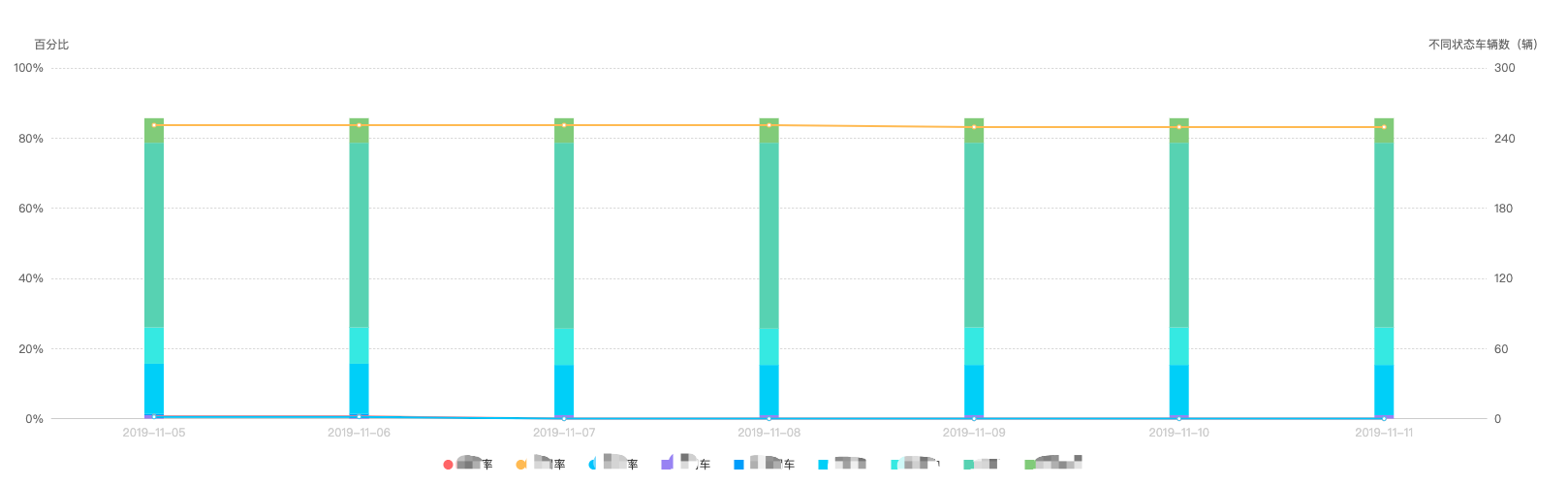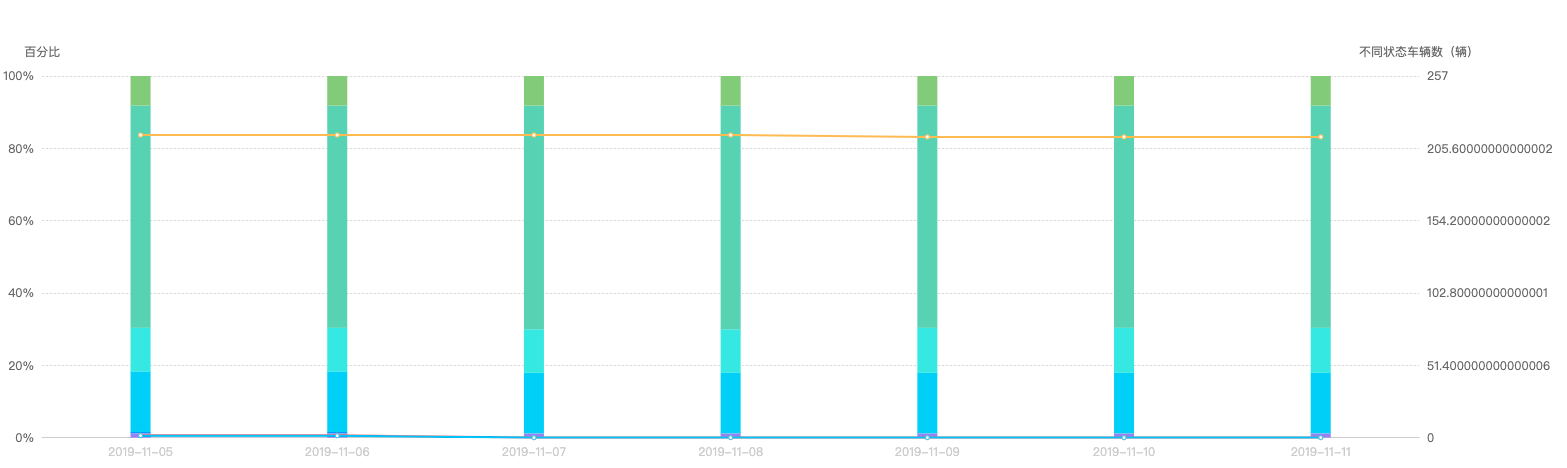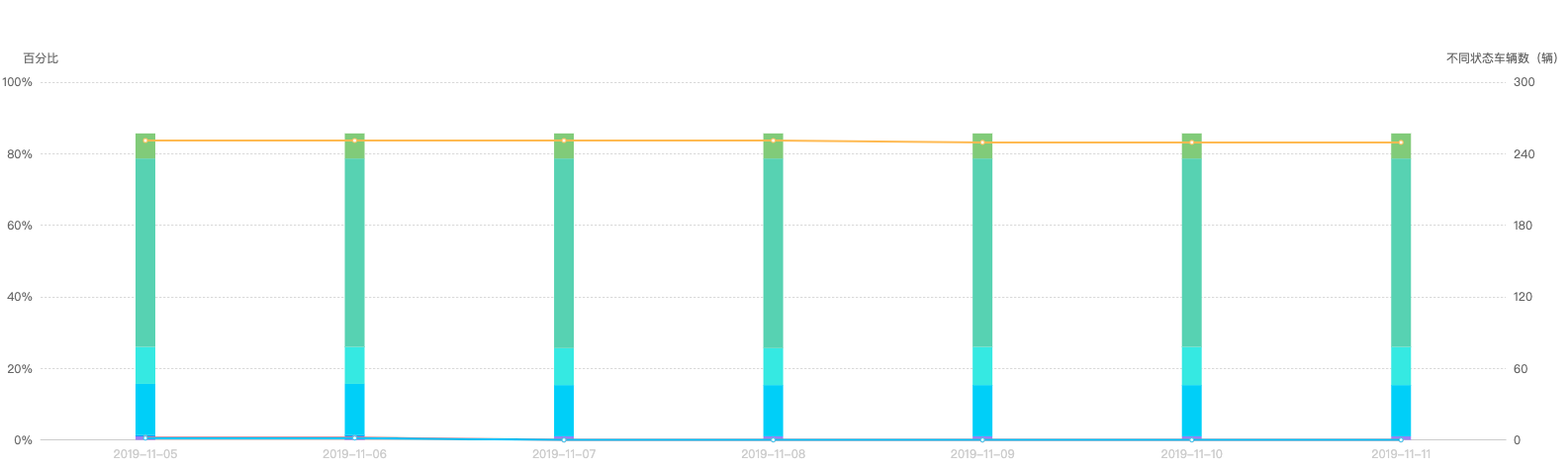# JS65 Echarts双Y轴刻度对齐

## 需求## 手动计算最大值

function getCeilMaxSumByColumn(arr = []) {
// 默认值
const DEFAULT_MAX = 100;
// 近似的间隔值
const IDEAL_INTERVAL = 50;
if (arr.length === 0) {
return DEFAULT_MAX;
}
// 按列求和，取最大值
const getMaxSumByColumn = arr => arr.reduce((result, current) = > {
current.forEach((num, day) = > {
if (!result[day]) {
result[day] = {
total: 0
};
}
result[day].total += num;
});
return result;
}, []).sort((a, b) => b.total - a.total).total;
//
const max = getMaxSumByColumn(arr);
if (max <= 0) {
return DEFAULT_MAX;
}
// 按照间隔
return Math.round(max);
},/**
* 获取二维数组按列求和的最大值，并按照 50 为分割，向上近似取值，例如输入
* [
*     [1, 2, 3],
*     [4, 5, 6],
*     [7, 8, 9]，
* ]
* 按列求和，第三列求和值最大为18，位于[0, 50]，输出结果为 50
* @param arr { array } 输入的二维数组
* @returns {number} 返回按列求和后的最大近似值
*/
function getCeilMaxSumByColumn(arr = []) {
// 默认值
const DEFAULT_MAX = 100;
// 近似的间隔值
const IDEAL_INTERVAL = 50;
if (arr.length === 0) {
return DEFAULT_MAX;
}
// 按列求和，取最大值
const getMaxSumByColumn = arr => arr.reduce((result, current) = > {
current.forEach((num, day) => {
if (!result[day]) {
result[day] = {
total: 0
};
}
result[day].total += num;
});
return result;
}, []).sort((a, b) = > b.total - a.total).total;
//
const max = getMaxSumByColumn(arr);
if (max <= 0) {
return DEFAULT_MAX;
}
// 按照间隔
return Math.ceil(max / IDEAL_INTERVAL) * IDEAL_INTERVAL;
},Done!

## 参考©️2019 CSDN 皮肤主题: 编程工作室 设计师: CSDN官方博客